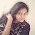# Time and Work: Techniques and examples with solutions

Today I'm going to discuss a very important topic i.e. Time and Work of quantitative aptitude. In almost every exam at least 2-3 question are asked every time. In this chapter, I will tell you about a definite relationship between Time and work and easy method to solve the problems.

## Three main factors of Time and Work

There is a definite relationship between Time and Work. In this concept, there are only three factors:
• Time taken to complete a certain job
• Unit of work done
• Number of persons doing the job
There is a fundamental relationship between these three, discussed as follows:

Work done(W) = Number of days(Time taken)(T or D) times Number of men(M)

W = D times M

### Some basic points

More number of men can do more work i.e. both are directly proportional
More number of men take less time to complete certain job i.e. both are inversely proportional

By summarizing, we get

(W_1)/(W_2) = ((M_1)/(M_2)) times ((D_1)/(D_2))

Lets start solving some examples:

Example1: 10 men can cut 8trees in 16 days. In how many days can 6men cut 10trees?

Solution: This is a very simple example. You are given:

W_1 = 8
W_2 = 10
M_1 = 10
M_2 = 6
D_1 = 16
D_2 = ?

Using formula,

(W_1)/(W_2) = ((M_1)/(M_2)) times ((D_1)/(D_2))

8/(10) = (10)/6 times (16)/(D_2)
⇒D_2 = 33.3

## Concept of efficiency

This means, "How much work one person can do in one day (expressed in percentage)"

For example: A person can do a job in 2days
⇒ He can do 50% work in one day
Therefore, his efficiency will be 50%

### Just a 2-step concept

This concept involves two steps to calculate efficiency:
• Convert into fraction i.e. per day work
• Multiply with 100 i.e. convert into percentage
Try the following example first, then re-read above points

Example2: If a person can complete his work in 5 days. What will be his efficiency?

Solution: Number of days a person take to complete his work = 5

⇒ He is doing 1/5 th work per day  (converted into fraction)

Convert it into percentage:

(100)/5 = 20%

Therefore, his efficiency is 20%.

Summarizing, If a person can do his job in n days, efficiency will be

Efficiency = (100)/n %

### Note: Negative efficiency cancels the positive efficiency

For Example: Positive efficiency = 5%
Negative efficiency = 1.5%
Net efficiency = 5- 1.5 = 3.5%

As we all know, in competitive exams time management is very important. I suggest you to learn the fractions till 15.

 Number of days required to complete work Work/Day Efficiency (%) N 1/n 100/n 1 1 100 2 1/2 50 3 1/3 33.33 4 1/4 25 5 1/5 20 6 1/6 16.66 7 1/7 14.28 8 1/8 12.5 9 1/9 11.11 10 1/10 10 11 1/11 9.09 12 1/12 8.33 13 1/13 7.69 14 1/14 7.14 15 1/15 6.66

Example3: A can do a job in 10 days. B can do a job in 5 days. In how many days they can complete the job if they work together?

Solution: Consider the above table

A's efficiency = 10% ((100)/(10))
B's efficiency = 20%

A+ B efficiency = 10 + 20 = 30%
This means, In one day A and B together can do 30% of work.

Therefore,Number of days A and B together take to do 100% of work = (100)/(30)

⇒3.33 days

Example4: A and B together can do a job in 4 days. If A can do job in 12 days if he works alone, then how many days B alone take to complete the job?

Solution: A+B take = 4 days

⇒ A+B's efficiency = 25% i.e. they together do 25% of work in one day

A takes = 12 days
⇒ A's efficiency = 8.33%

B's efficiency = (A+B) - (A)

⇒ 25% - 8.33% = 16.66%

This means, B can do 16.66% work in one day
Therefore, to complete the job he will take = (100)/(16.66) days
⇒ 6days

Example5: A and B can do job in 8 days. B and C can do same job in 12 days. A, B and C together can do same job in 6 days. In how many days A and C together can complete the job?

Solution: You are given that:

A+B's efficiency = 12.5%
B+C's efficiency = 8.33%
A+B+C's efficiency = 16.66%

we need to find A+C

Consider, 2(A+B+C) = (A+B) + (B+C) + (C+A)
⇒2(16.66) = 12.5+ 8.33 + (C+A)
⇒ C+A = 12.49 = 12.5%
Therefore, A and C takes= (100)/(12.5) = 8 days

Hope you all understand this topic. I will soon update questions for your practice.

#### What's trending in BankExamsToday

Smart Prep Kit for Banking Exams by Ramandeep Singh - Download here1.thank u :)

2.thanku so much these techniques are easy...hope they will be helpfull to me in exams...once again thanku sooo much sir..

3.Superb Explanation Sir....Thank you so much

4.thnx evryone for appreciating,,, but pls call me mam not sir...

5.6.Dear Mam,
The value of efficiency of 12th day will be 8.33, Please correct it.

7.डियर मैडम जी,
वो 12th दिन की ईफीसीएंसी वैल्यू 8.33 होगी, क्रप्या सुधार करें!

I will try to respond asap